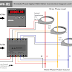Hey, in this article we are going to see the connection diagram of 3 phase digital kWh meter. Here, we will see how to connect the CT with a kWh meter. A kWh meter is basically a measuring instrument that is used to measure the electrical energy in kWh. kWh means Kilo Watt Hour. That means it helps us to measure how much kilo-watt power is consumed per 1 hour. This type of digital kWh meter is used in industrial panels to measure how much electricity is consumed by the load connected to that panel. These are also known as panel meters. This type of Digital kWh meter not only shows the energy consumption but we can also see the below parameters,
1. Active Energy
2. Active Energy Old
3. Apparent Energy
4. Apparent Energy Old
5. Power Factor
6. Apparent Power
7. Power Factor R-phase
8. Power Factor Y-phase
9. Power Factor B-phase
10. Watts R-phase
11. Watts Y-phase
12. Watts B-phase
13. Watts Total
14. Apparent Power R-phase
15. Apparent Power Y-phase
16. Apparent Power B-phase

## Three(3) Phase Digital kWh Meter Connection Diagram

Here, you can see the connection diagram of Three(3) Phase Digital kWh Meter.

Connection Procedure
1. Connect 230V AC or 110V AC Auxiliary power supply to the kWh meter as per your meter requirement. You can connect the auxiliary power supply from an external power source or from the existing three-phase power source by taking any one phase and neutral. But in this case, your meter must be designed for a 230V AC auxiliary power supply.
2. Now connect all the three-phase and neutral lines from the three-phase power source( that is used to drive the load) to the kWh meter voltage input terminals. These terminals may be identified as R, Y, B, or N or L1, L2, L3, or N. Make all the connections as per the identification, don't interchange any phase or neutral.
3. Ensure that all the three-phase lines such as R, Y, and B go through their respective CTs such as CT1, CT2, and CT3.
4. Now connect all the CTs to the kWh meter CT terminals. Here in the above diagram, they are identified as A1 for CT1, A2 for CT2, and A3 for CT3.
5. Once you have completed and verified all the connections, you can turn on the power to the circuit. The kWh meter should now start measuring the electricity consumption on the designated circuit. Some digital panel kWh meters may require calibration or programming to match the specific electrical parameters of the circuit. Consult the manufacturer's instructions for any calibration or programming steps that need to be performed.

The CT (Current Transformer) ratio is crucial for kWh meter connections because it ensures accurate measurement and monitoring of electrical energy consumption. The primary purpose of a CT is to step down the current flowing through a circuit to a level suitable for the kWh meter to measure. The CT ratio determines the relationship between the actual current in the circuit and the reduced current measured by the kWh meter. It allows the meter to accurately reflect the real current consumption.

It is recommended to take the same current ratio for both CT and kWH meters such as 100:5 for CT and 5:100 for kWh Meter. By using the same current ratio, you eliminate the need for additional calculations or adjustments to scale the secondary current of the CT to match the primary current input range of the kWh meter. This simplifies the configuration process.

Thank you for visiting the Website. Keep visiting for more Updates.

Three(3) Phase Digital Panel kWh Meter Connection DiagramReviewed by Author on June 09, 2023 Rating: 5